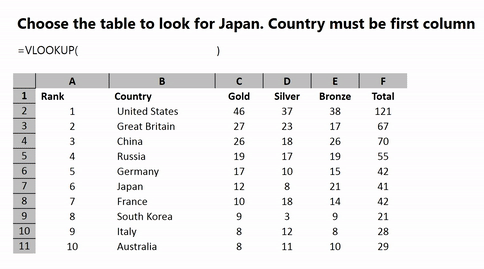# VLOOKUP for DummiesVLOOKUP stands for Vertical Lookup. Learning vlookup is very easy but let’s first understand how VLOOKUP works? Even dummies can easily do VLOOKUP in Excel. In plain English VLOOKUP is:

- Go down until you find X in the first column;

- When you find X, then go right Y columns

- Tell me the value

### Here is a VLOOKUP Example in Excel

Tell me how many silver medals did JAPAN get in 2016 Summer Olympics?Do you need more practice about VLOOKUP? Here are a few examples that you can do;

### Some rules;

- VLOOKUP search returns the first match

- VLOOKUP always goes to right, never left

- VLOOKUP is not case sensitive  (See Case Sensitive Lookup)

- VLOOKUP has two mode, exact matach or approximate match. Default is approximate! Be careful to set it exact if needed.  (See Exact Match)

- VLOOKUP needs mostly an absolute reference for the lookup table. (See  Cell referencing section)

- VLOOKUP return #N/A if no match, you can check it with IFNA() function (See Lookups)

- Numbers stored as Text can cause VLOOKUP to give #N/A error

- VLOOKUP stand alone can take only one criteria. (See How to do Multiple Criteria VLOOKUP)

Also take look at our Master Lookups course

### Mispelling VLOOKUP

There are various errors made while writing VLOOKUP. So never use V LOOKUP, V LOOK UP or VLOOK UP. All is one word VLOOKUP.